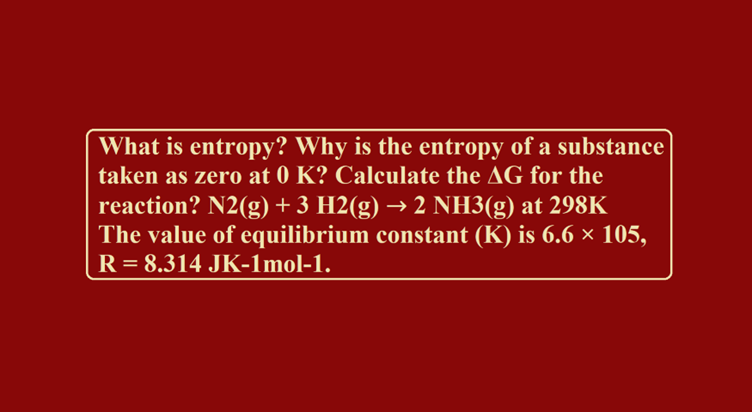# What is entropy? Why is the entropy of a substance taken as zero at 0K? Calculate the ΔG for the reaction? N2(g) + 3H2(g) → 2NH3(g) at 298K The value of equilibrium constant (K) is 6.6 × 105, R = 8.314 JK-1mol-1.Que. What is entropy? Why is the entropy of a substance taken as zero at 0 K? Calculate the ΔG for the reaction? N2(g) + 3 H2(g) → 2 NH3(g) at 298K
The value of equilibrium constant (K) is 6.6 × 105, R = 8.314 JK-1mol-1.
Ans. It is measure of randomness or disorder of system. Because at 0K there is complete order in the system.
ΔG0 = – 2.303 RT log K = – 2.303 × 8.314 × 298 × log 6.6 × 105
= – 5705.8 [log 6.6 + log 105]
= – 5705.8 [0.8195 + 5.0] = – 5705.8 × 5.8195 J
= – 33204.903 J
ΔG0 = – 33.205 KJ mol-1.